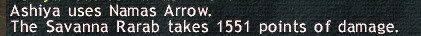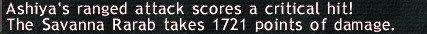- the StarOnion – FFXI Fenrir to FFXIV Excalibur - https://www.staronion.com/maiev/nfblog -

# Yoichinoyumi Test #1.

1st MythYoichinoyumi has no DMG cap:

When people talk about DMG cap, they usually mean fSTR2 cap. You cannot change the maximum Weapon’s damage + Ammo damage, as well as the maximum pDiF (3 non-critical; 3.75 critical). So only fSTR2 is the variable factor when everything is capped in the damage formula:

Damage = floor((WD + AD + fSTR2) x pDiF)

Test on Low level monsters in West Sarutabaruta, which means pDiF is capped at 3. For the test of DMG cap, I used Yoichinoyumi + Kabura Arrows, which give Weapon’s damage + Ammo damage = 81 + 38 = 119

If fSTR2 is capped, it should be : fSTR2 = (Weapon’s rank + 8 ) x 2 = (floor(81/9) + 8 ) x 2 = 34

That means:

• Normal shot should be: (119+34) x 3 = 459
• Normal shot with piercing bonus 25%: (119+34) x 3 x 1.25 = 573Results from the test agree with the preliminary assumptions, which means Yoichinoyumi does have an fSTR2 cap.

Myth busted!

2nd Myth – Namas Arrow has WSC: 40% AGI, 40% STR; fTP = 2.75

In WS gears for the test, I have 96 STR (was /BLM >.>) and 112 AGI in total:

WSC = floor(floor((0.4×112+0.4×96)x0.83)) = 68

Damage = floor(floor((WD + AD + fSTR2 + 68) x 2.75) x 3)

Plug-in the number: For this test, I used Yoichinoyumi + Wooden Arrows, so total WD + AD = 81 + 5 = 86; fSTR2 should be capped from above test, so fSTR2 = 34; thus Namas damage should be:

Namas = floor(floor((86 + 34 + 68) x 2.75) x 3) = 1551Results from the 2nd test agree with this preliminary assumptions.

Myth confirmed!

Noted that the result was correct with the main assumption of fTP to be 2.75; if fTP is different, then theoretically, the WSC on Namas Arrow could be different, but since the result is exact to the last digit, I believe both fTP and WSC of Namas in the assumption are correct.

————————————————————-

An extra: This is the highest single shot damage that you can ever get with Yoichinoyumi, w/o PPA (or piercing bonus) :D